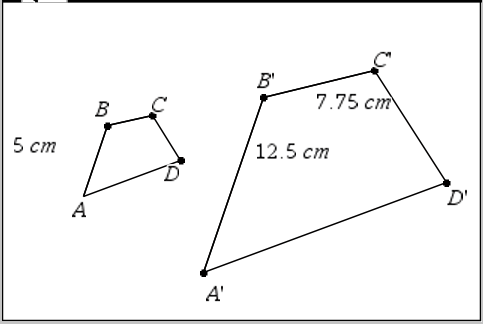# Ratios and Proportions / Connecting Ratios and Scaling

Activity 14 of 15
In this lesson, students investigate how scale factors are used to make figures larger or smaller, depending on whether the ratio is greater or less than 1.

## Planning and Resources

Objectives
Students should understand how sides of a figure are related by a scale factor. They can identify the scale factor given that one figure has been scaled from another.

Vocabulary
scale factor

Standard:

## Lesson Snapshot

#### Understanding

When a figure is scaled, the ratios between the corresponding sides of the two figures are equivalent, and the internal ratios between two sides of one figure are equivalent to the internal ratio for the corresponding sides of the second figure.

### What to look for

Students should note the relationship between the corresponding sides of two scaled figures; that a scale factor relates the sides of one figure to the sides of the other.

### Sample Assessment

Figure A′B′C′D′ has been scaled from figure ABCD.a. What is the scale factor? Answer: 2.5

b. What is length BC? Answer: 3.1

#### The Big Idea

When a figure is scaled, the ratios between the corresponding sides of the two figures are equivalent, and the internal ratios between two sides of one figure are equivalent to the internal ratio for the corresponding sides of the second figure.

### What are the students doing?

Students use the relationship between the sides of two scaled figures and the scale factor to find missing dimensions in the scaled figure and to solve problems involving scale factors.

### What is the teacher doing?

Scaling can be associated with similar figures, but this lesson is an informal introduction to the ideas related to similarity and the language of similarity is not introduced here.